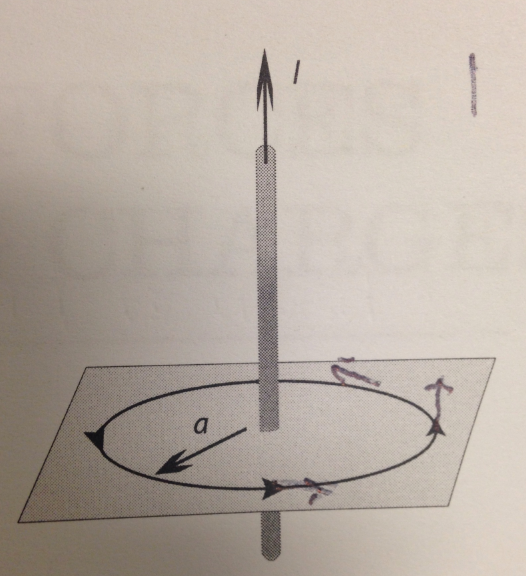###### FREE Expert Solution

Remember the geographic north pole is actually a magnetic south—that's why the north pole of a compass needle points to it.

Use right-hand rule to find direction of wire's magnetic field: thumb in direction of current, fingers curl in the direction of Bwire.

Magnitude of magnetic field from long straight current-carrying wire:

$\overline{){\mathbit{B}}{\mathbf{=}}\frac{{\mathbit{\mu }}_{\mathbf{0}}\mathbit{I}}{\mathbf{2}\mathbit{\pi }\mathbit{R}}}$

where μ0 = 4π×10−7 T·m/A.

The intensity of the earth's magnetic field is 25 μT - 65 μT (2.5×10−5 T - 6.5×10−5 T).

92% (285 ratings)###### Problem Details

MEASURING EARTH'S MAGNETIC FIELD

The goal of this section of the lab is to measure the Earth's magnetic field. Since the β-field produced by the Earth is a vector, it has a magnitude and a direction. You have already measured the direction of the Earth's β-field, so all that is left to measure is its magnitude. By introducing another magnetic field (produced by the coil of wire attached to the wooden box) and using the compass, you will be able to measure the total magnetic field (Earth and wire fields combined), and use that information to infer the magnitude of the Earth's field alone.

Practical considerations restrict the current to about 2.0 A. To overcome this we have multiple wires running parallel to each other, each carrying about 2.0 A. This setup also has a platform where you can place a sheet of paper and a compass. You can mark the angle of the compass needle on the paper.

E1. Before you connect the loops of wire to the supply, set the current limit of the supply to its maximum value, and set the voltage to 2.5 V. ( This is just to make sure there is not too much current in the wires when you turn the supply on.) Then switch the supply to read current, and turn the power supply off. Cut a sheet of paper to fit around the wire and rest on the platform to record the direction of the magnetic field. Set up the circuit so that the "conventional" current is flowing upwards.

E2. Test your setup: turn the power supply on and verify that you get a current of about 2.0 A. (This may not correspond to 2.5 V, which is ok) Do not leave the supply for about 2.0 A. (This may correspond to 2.5 V, which is ok) Do not leave the supply on for more than 10 seconds to avoid overheating. Note the current and the number of wires in your setup.

DATA (3):

(a) Draw a top-down diagram of your setup of image provided: I is current passing through wire; arrows are vectors of compass pointing. This diagram should include:

- The direction of current in the wires

- The direction of the compass needle

- Vectors representing the Earth's magnetic field and the magnetic field of the wire at the location of the compass

- Known and relevant distances, angles, and currents

- The cardinal directions (N,S,E,W)

Use your measurements from part E to calculate the strength of the Earth’s magnetic field. Show your work.

Measurement: 2.5-A current through wire pointing upwards (down to up), an angle the needle makes on compass (north pointing towards wire) when current is 2.5: 37° +/- 3°. Distance of needle of compass to wire: (2.1 +/- 0.5) cm. hint: tanθ = Bwire/BEarth.

Calculate the uncertainty in your measurement of the Earth's magnetic field.

Compare your measured magnetic field and uncertainty to the official range of values for the Earth's surface magnetic field. Are your measurements consistent with the official values?